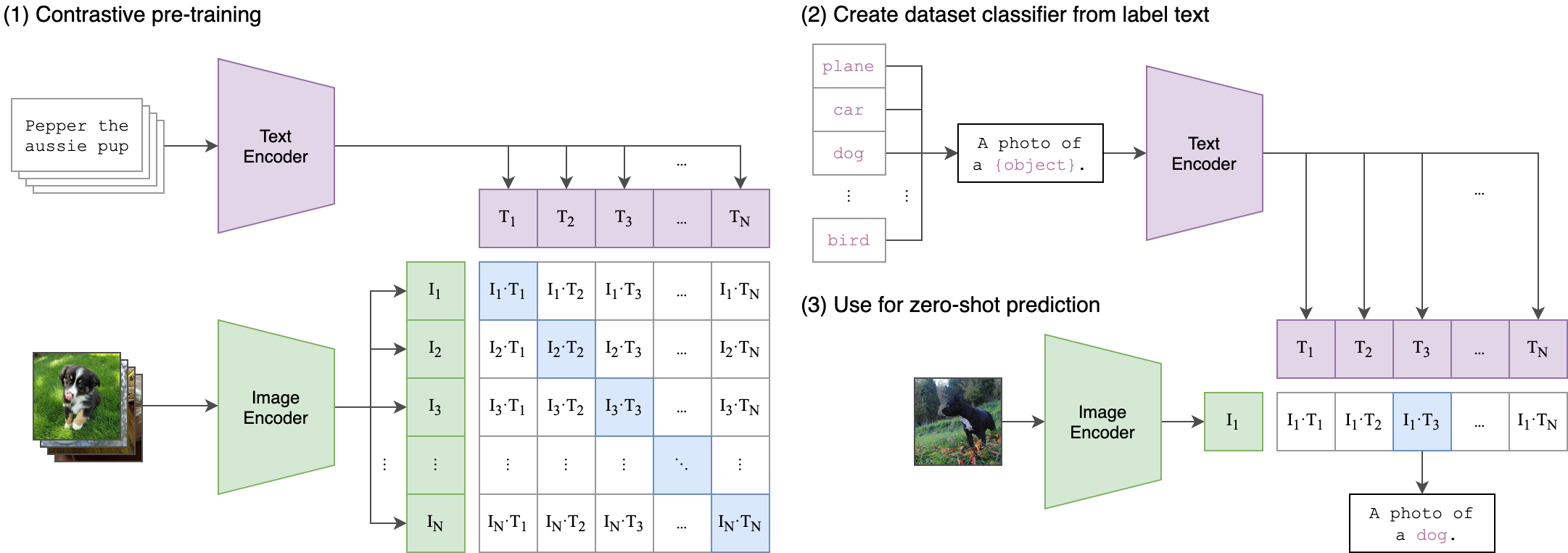# CauchyFood / CLIP

Contrastive Language-Image Pretraining

Geek Repo

Github PK Tool

# CLIP

CLIP (Contrastive Language-Image Pre-Training) is a neural network trained on a variety of (image, text) pairs. It can be instructed in natural language to predict the most relevant text snippet, given an image, without directly optimizing for the task, similarly to the zero-shot capabilities of GPT-2 and 3. We found CLIP matches the performance of the original ResNet50 on ImageNet “zero-shot” without using any of the original 1.28M labeled examples, overcoming several major challenges in computer vision.

## Approach## Usage

First, install PyTorch 1.7.1 and torchvision, as well as small additional dependencies, and then install this repo as a Python package. On a CUDA GPU machine, the following will do the trick:

```\$ conda install --yes -c pytorch pytorch=1.7.1 torchvision cudatoolkit=11.0
\$ pip install ftfy regex tqdm
\$ pip install git+https://github.com/openai/CLIP.git```

Replace `cudatoolkit=11.0` above with the appropriate CUDA version on your machine or `cpuonly` when installing on a machine without a GPU.

```import torch
import clip
from PIL import Image

device = "cuda" if torch.cuda.is_available() else "cpu"

image = preprocess(Image.open("CLIP.png")).unsqueeze(0).to(device)
text = clip.tokenize(["a diagram", "a dog", "a cat"]).to(device)

image_features = model.encode_image(image)
text_features = model.encode_text(text)

logits_per_image, logits_per_text = model(image, text)
probs = logits_per_image.softmax(dim=-1).cpu().numpy()

print("Label probs:", probs)  # prints: [[0.9927937  0.00421068 0.00299572]]```

## API

The CLIP module `clip` provides the following methods:

#### `clip.available_models()`

Returns the names of the available CLIP models.

#### `clip.load(name, device=..., jit=False)`

Returns the model and the TorchVision transform needed by the model, specified by the model name returned by `clip.available_models()`. It will download the model as necessary. The `name` argument can also be a path to a local checkpoint.

The device to run the model can be optionally specified, and the default is to use the first CUDA device if there is any, otherwise the CPU. When `jit` is `False`, a non-JIT version of the model will be loaded.

#### `clip.tokenize(text: Union[str, List[str]], context_length=77)`

Returns a LongTensor containing tokenized sequences of given text input(s). This can be used as the input to the model

The model returned by `clip.load()` supports the following methods:

#### `model.encode_image(image: Tensor)`

Given a batch of images, returns the image features encoded by the vision portion of the CLIP model.

#### `model.encode_text(text: Tensor)`

Given a batch of text tokens, returns the text features encoded by the language portion of the CLIP model.

#### `model(image: Tensor, text: Tensor)`

Given a batch of images and a batch of text tokens, returns two Tensors, containing the logit scores corresponding to each image and text input. The values are cosine similarities between the corresponding image and text features, times 100.

## More Examples

### Zero-Shot Prediction

The code below performs zero-shot prediction using CLIP, as shown in Appendix B in the paper. This example takes an image from the CIFAR-100 dataset, and predicts the most likely labels among the 100 textual labels from the dataset.

```import os
import clip
import torch
from torchvision.datasets import CIFAR100

device = "cuda" if torch.cuda.is_available() else "cpu"

# Prepare the inputs
image, class_id = cifar100
image_input = preprocess(image).unsqueeze(0).to(device)
text_inputs = torch.cat([clip.tokenize(f"a photo of a {c}") for c in cifar100.classes]).to(device)

# Calculate features
image_features = model.encode_image(image_input)
text_features = model.encode_text(text_inputs)

# Pick the top 5 most similar labels for the image
image_features /= image_features.norm(dim=-1, keepdim=True)
text_features /= text_features.norm(dim=-1, keepdim=True)
similarity = (100.0 * image_features @ text_features.T).softmax(dim=-1)
values, indices = similarity.topk(5)

# Print the result
print("\nTop predictions:\n")
for value, index in zip(values, indices):
print(f"{cifar100.classes[index]:>16s}: {100 * value.item():.2f}%")```

The output will look like the following (the exact numbers may be slightly different depending on the compute device):

``````Top predictions:

snake: 65.31%
turtle: 12.29%
sweet_pepper: 3.83%
lizard: 1.88%
crocodile: 1.75%
``````

Note that this example uses the `encode_image()` and `encode_text()` methods that return the encoded features of given inputs.

### Linear-probe evaluation

The example below uses scikit-learn to perform logistic regression on image features.

```import os
import clip
import torch

import numpy as np
from sklearn.linear_model import LogisticRegression
from torchvision.datasets import CIFAR100
from tqdm import tqdm

device = "cuda" if torch.cuda.is_available() else "cpu"

root = os.path.expanduser("~/.cache")

def get_features(dataset):
all_features = []
all_labels = []

for images, labels in tqdm(DataLoader(dataset, batch_size=100)):
features = model.encode_image(images.to(device))

all_features.append(features)
all_labels.append(labels)

# Calculate the image features
train_features, train_labels = get_features(train)
test_features, test_labels = get_features(test)

# Perform logistic regression
classifier = LogisticRegression(random_state=0, C=0.316, max_iter=1000, verbose=1)
classifier.fit(train_features, train_labels)

# Evaluate using the logistic regression classifier
predictions = classifier.predict(test_features)
accuracy = np.mean((test_labels == predictions).astype(np.float)) * 100.
print(f"Accuracy = {accuracy:.3f}")```

Note that the `C` value should be determined via a hyperparameter sweep using a validation split.